# GED Math : Basic Operations

## Example Questions

← Previous 1 3 4 5 6 7 8 9 16 17

### Example Question #1 : Addition And Subtraction

Identify the property of addition demonstrated by the following statement: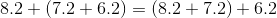Explanation:

The statement shows that the sum of three numbers yields the same result regardless of the order in which they are added; this is a demonstration of the associative property of addition.

### Example Question #1 : Addition And Subtraction

Identify the property of addition demonstrated by the following statement: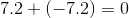Explanation:

The statemet shows a number being added to its additive inverse to obtain a sum of 0; this is a demonstration of the inverse property of addition.

### Example Question #3 : Basic Operations

Identify the property of addition demonstrated by the following statement: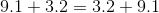Explanation:

This statement shows that two numbers can be added in either order to achieve the same result; this is a demonstration of the commutative property of addition.

### Example Question #161 : Numbers And Operations

Forty-two friends are renting a party bus to the school prom. Rental of the party bus will cost $320 up front plus$18 per hour. The friends have the bus from 5:00 PM to 3:00 AM.

Each friend has agreed to chip in $9. One of the teachers at the high school, Mr. Smith, has offered to chip in the rest. If he does, how much will he pay? Possible Answers: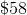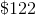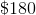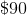Correct answer:Explanation: The friends have the bus from 5:00 PM to 3:00 AM - this is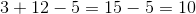hours. The cost of renting the bus will be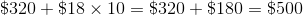. Forty-two friends paying$9 each will pay a total of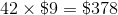.

The teacher will pay the difference, or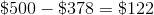.

### Example Question #1 : Basic Operations

A plane takes off at Cheyenne Regional Airport, which has an elevation of 6,159 feet. It ascends 17,785 feet, then descendsfeet. Which expression gives the current elevation of the plane in feet?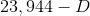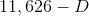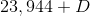Explanation:

The initial elevation of the plane is 6,159 feet in elevation. Ascent is an increase in elevation and descent is a decrease, so, to the elevation, in turn, add 17,785 feet,  and subtractfeet. This is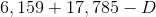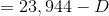### Example Question #1 : Addition And Subtraction

An airplane takes off from Denver International Airport, which has elevation 5,431 feet. It is now flying at an elevation of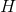feet. How many feet has the airplane ascended since takeoff?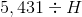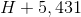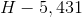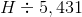Explanation:

We are looking for the difference between the current elevation of the plane and the initial elevation, so we subtract the initial elevation, 5,431, from current elevation,. This is.

### Example Question #2 : Addition And Subtraction

Identify the property of addition demonstrated by the following statement: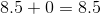Explanation:

0 is called the additive identity in that it can be added to any number to yield that other number as the sum.

The identity property of addition is written mathematically as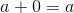for any real.

### Example Question #171 : Ged Math

Add the numbers: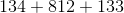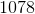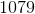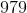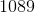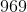Explanation: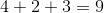Add the tens digits.  There is no carryover.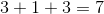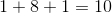Combine this number with the other digits.

The answer is:### Example Question #1 : Addition And Subtraction

Add the numbers: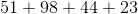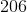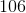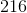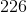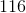Explanation: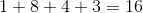Add the tens digits with the one as the carryover.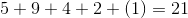Combine this number with the ones digit of the first number.

The answer is:### Example Question #1 : Addition And Subtraction

Add the numbers: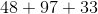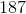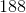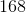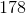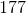Explanation: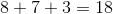Add the tens digits with the 1 as the carryover from the last calculation.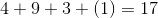Combine this number with the ones digit of the first calculation.

The answer is:← Previous 1 3 4 5 6 7 8 9 16 17

### All GED Math Resources